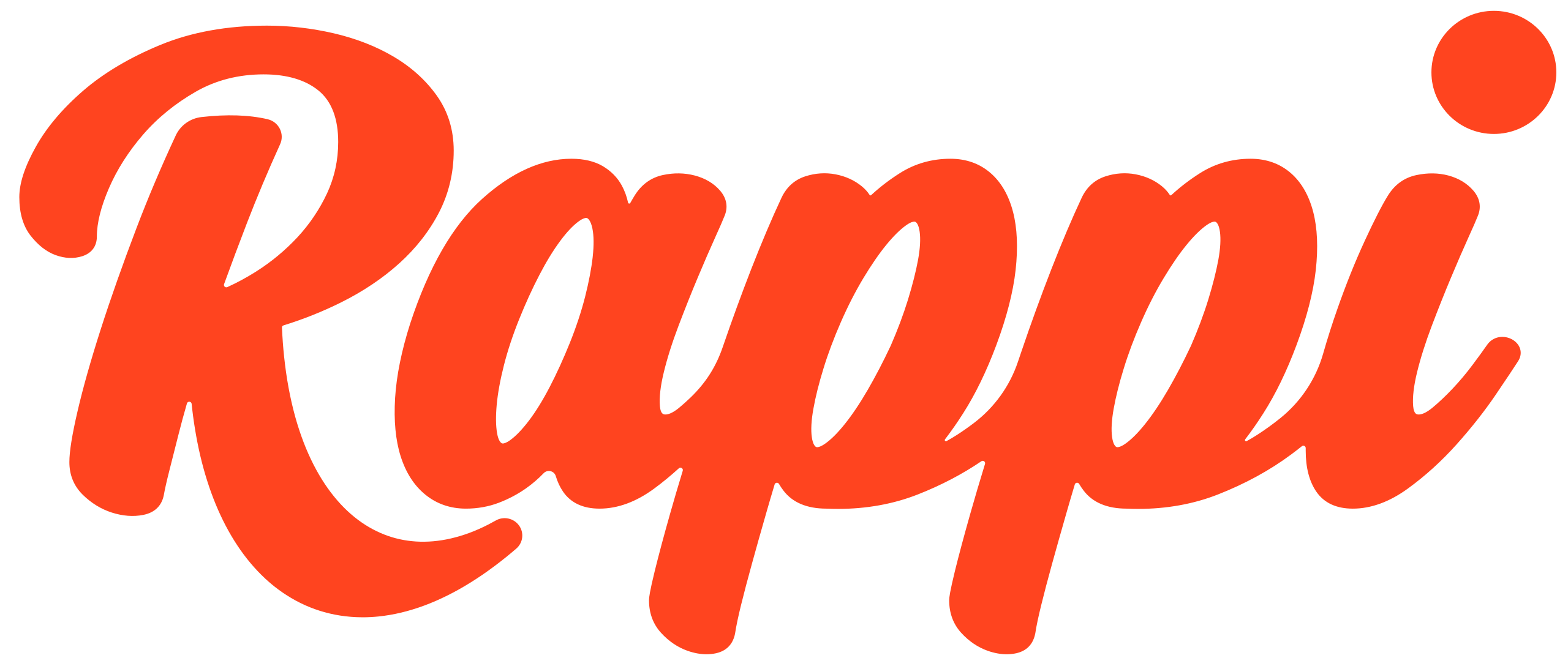# Statistics & Probability

Covers descriptive and inferential statistics, probability concepts, correlation and regression analysis, and applying statistics and probability to analytics projects.4.8

/

5

Data Analytics

Difficulty

Hours per week

Live Sessions

## Sep 24, 2023

Next Date

Objective

The main objective of this course is to equip you with the necessary knowledge and skills to interpret data using statistical methods and probability concepts. By the end of the course, you will have a better understanding of data analysis, hypothesis testing, and probability distribution.

# Requeriments

• Familiarity with basic mathematical concepts and operations.

• Proficiency in algebraic calculations and equations.

• Basic understanding of data analysis concepts and terminology.

• Basic familiarity with statistical concepts such as variables, populations, samples, and probability.

• Curiosity and eagerness to apply statistical and probability concepts to data analytics projects for informed decision-making and predictive analysis.

# Syllabus

1
Descriptive Statistics

Learn to summarize and describe data using descriptive statistics. Explore measures of central tendency such as the mean, median, and mode, which provide insights into the typical values in a dataset. Measures of dispersion, including the range, variance, and standard deviation, will be covered to understand the spread and variability of the data. Apply visualization techniques like histograms, box plots, and scatter plots, to gaining a visual understanding of the data distribution and relationships.

2
Inferential Statistics

Draw conclusions and make predictions about a population based on a sample of data. Learn about hypothesis testing, for making decisions and determining the significance of relationships in the data, and explore confidence intervals, analysis of variance (ANOVA), and the chi-square test to analyze and compare data from different groups and variables.

3
Probability Concepts

Probability is the foundation of statistical reasoning. Undestand fundamental concepts of probability, including sample spaces and events. Explore random variables, which represent uncertain outcomes in a probabilistic context. Probability distributions, such as binomial, normal, and Poisson distributions, will be studied to understand the likelihood of various events occurring. Gain insights into Bayes' theorem and its relevance in conditional probability and Bayesian inference, which is a powerful tool for updating probabilities based on new information.

4
Correlation and Regression

Correlation and regression are essential techniques for analyzing the relationship between variables. Learn about correlation coefficients, specifically Pearson, which quantify the strength and direction of linear relationships between two variables. Linear regression will be explored as a method to model and predict the dependent variable. Understanding these concepts to identify and interpret relationships between variables in real-world datasets, making predictions and drawing meaningful conclusions.

# Mentor

Mentor to be defined.
Our alumni works in:# Learn all you can. No extra fees, no commissions, no surprises.

— We’re an hybrid learning platform with live-cohorts. Learn everything you want by acquiring a membership.